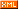# 生活如同楼梯般的上上下下

:: :: :: :: :: :::: ::13 随笔 :: 0 文章 :: 28 评论 :: 0 引用

Expression<Func<UserInfo, bool>> where;

ParameterExpression param = Expression.Parameter(typeof(UserInfo), "c");//c=>

//c=>c.IsDelete==false这里需要不被删除的条件

MemberExpression left1 = Expression.Property(param, typeof(UserInfo).GetProperty("IsDelete"));构建c.IsDelete

ConstantExpression right1 = Expression.Constant(false);//构建一个常量 false

BinaryExpression be = Expression.Equal(left1, right1);构建//c=>c.IsDelete==false 就是现在这个be了

if (!string.IsNullOrEmpty(Request["sid"]))

{

//c.UserId==sid

int sid = int.Parse(Request["sid"]);

//根据参数的属性构造左表达式c.UserId

MemberExpression left2 = Expression.Property(param, typeof(UserInfo).GetProperty("UserId"));

//构造右表达式sid

ConstantExpression right2 = Expression.Constant(sid);

//进行合并：cUserId==sid

BinaryExpression where2 = Expression.Equal(left2, right2);

//将这个条件与上一个条件进行与合并：c.IsDelete==false && c.UserId==sid

be = Expression.And(be, where2);

}

 然后看了示例这个 究竟是用来干嘛的??

if (!string.IsNullOrEmpty(Request["sname"]))

{

string sname = Request["sname"];

ConstantExpression right3 = Expression.Constant(sname);//这里构造sname这个常量表达式

MethodCallExpression where3 = Expression.Call(left3, typeof(string).GetMethod("Contains"), right3);这里我们用Call这个方法完成/c.UserName.Contains(sname)这个lambda这个表达式的实现.

be = Expression.And(be, where3);//拼接刚才的be表达式,

}

where = Expression.Lambda<Func<UserInfo, bool>>(be, param);//生成最后需要的带参数的表达式树.

public class WhereHelper<T>

where T:class

{

private ParameterExpression param;

private BinaryExpression filter;

public WhereHelper()

{

param = Expression.Parameter(typeof (T), "c");

//1==1

Expression left = Expression.Constant(1);

filter = Expression.Equal(left, left);

}

public Expression<Func<T, bool>> GetExpression()

{

return Expression.Lambda<Func<T, bool>>(filter,param);

}

public void Equal(string propertyName,object value)

{

Expression left = Expression.Property(param, typeof (T).GetProperty(propertyName));

Expression right = Expression.Constant(value, value.GetType());

Expression result = Expression.Equal(left, right);

filter = Expression.And(filter, result);

}

public void Contains(string propertyName,string value)

{

Expression left = Expression.Property(param, typeof (T).GetProperty(propertyName));

Expression right = Expression.Constant(value, value.GetType());

Expression result = Expression.Call(left, typeof (string).GetMethod("Contains"), right);

filter = Expression.And(filter, result);

}

}

posted on 2014-06-06 20:37  生活如同楼梯般的上上  阅读(...)  评论(...编辑  收藏